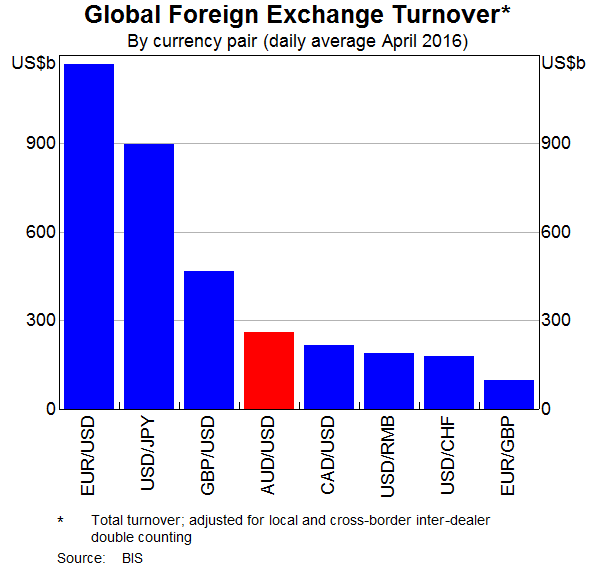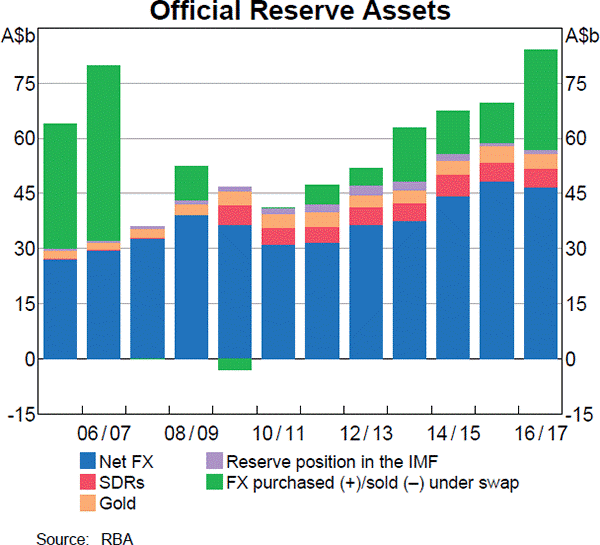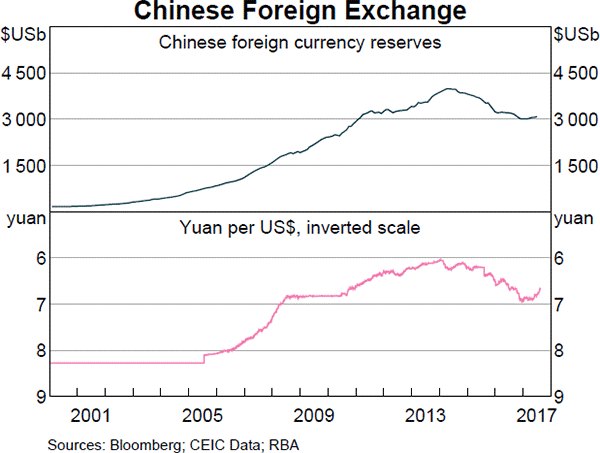# Fight Finance

#### CoursesTagsRandomAllRecentScores

A stock is expected to pay the following dividends:

 Cash Flows of a Stock Time (yrs) 0 1 2 3 4 ... Dividend ($) 0.00 1.00 1.05 1.10 1.15 ... After year 4, the annual dividend will grow in perpetuity at 5% pa, so; • the dividend at t=5 will be$1.15(1+0.05),
• the dividend at t=6 will be $1.15(1+0.05)^2, and so on. The required return on the stock is 10% pa. Both the growth rate and required return are given as effective annual rates. What will be the price of the stock in three and a half years (t = 3.5)? A wholesale building supplies business offers credit to its customers. Customers are given 60 days to pay for their goods, but if they pay within 7 days they will get a 2% discount. What is the effective interest rate implicit in the discount being offered? Assume 365 days in a year and that all customers pay on either the 7th day or the 60th day. All rates given below are effective annual rates. In Germany, nominal yields on semi-annual coupon paying Government Bonds with 2 years until maturity are currently 0.04% pa. The inflation rate is currently 1.4% pa, given as an APR compounding per quarter. The inflation rate is not expected to change over the next 2 years. What is the real yield on these bonds, given as an APR compounding every 6 months? A very low-risk stock just paid its semi-annual dividend of$0.14, as it has for the last 5 years. You conservatively estimate that from now on the dividend will fall at a rate of 1% every 6 months.

If the stock currently sells for $3 per share, what must be its required total return as an effective annual rate? If risk free government bonds are trading at a yield of 4% pa, given as an effective annual rate, would you consider buying or selling the stock? The stock's required total return is: Question 472 quick ratio, accounting ratio A firm has current assets totaling$1.5b of which cash is $0.25b and inventories is$0.5b. Current liabilities total $2b of which accounts payable is$1b.

What is the firm's quick ratio, also known as the acid test ratio?

Which of the following FX quotes (current in October 2015) is given in American terms?

Alice, Bob, Chris and Delta are traders in the futures market. The following trades occur over a single day in a newly-opened equity index future that matures in one year which the exchange just made available.

1. Alice buys a future from Bob.

2. Chris buys a future from Delta.

3. Alice buys a future from Chris.

These were the only trades made in this equity index future. What was the trading volume and what is the open interest?

Fred owns some Commonwealth Bank (CBA) shares. He has calculated CBA’s monthly returns for each month in the past 20 years using this formula:

$$r_\text{t monthly}=\ln⁡ \left( \dfrac{P_t}{P_{t-1}} \right)$$

He then took the arithmetic average and found it to be 1% per month using this formula:

$$\bar{r}_\text{monthly}= \dfrac{ \displaystyle\sum\limits_{t=1}^T{\left( r_\text{t monthly} \right)} }{T} =0.01=1\% \text{ per month}$$

He also found the standard deviation of these monthly returns which was 5% per month:

$$\sigma_\text{monthly} = \dfrac{ \displaystyle\sum\limits_{t=1}^T{\left( \left( r_\text{t monthly} - \bar{r}_\text{monthly} \right)^2 \right)} }{T} =0.05=5\%\text{ per month}$$

Which of the below statements about Fred’s CBA shares is NOT correct? Assume that the past historical average return is the true population average of future expected returns.

Which of the following statements is NOT correct? Fairly-priced assets should:

Examine the below graphs. The first graph shows daily FX turnover in the world by both the public (government) and private sectors. The second graph 'Official Reserve Assets' shows the FX reserves of the Australian central bank, the RBA. The third graph's top panel shows the FX reserves of the Chinese central bank, the PBoC.Assume that the AUD and USD are priced at parity so 1 AUD = 1 USD.

Which of the following statements is NOT correct?